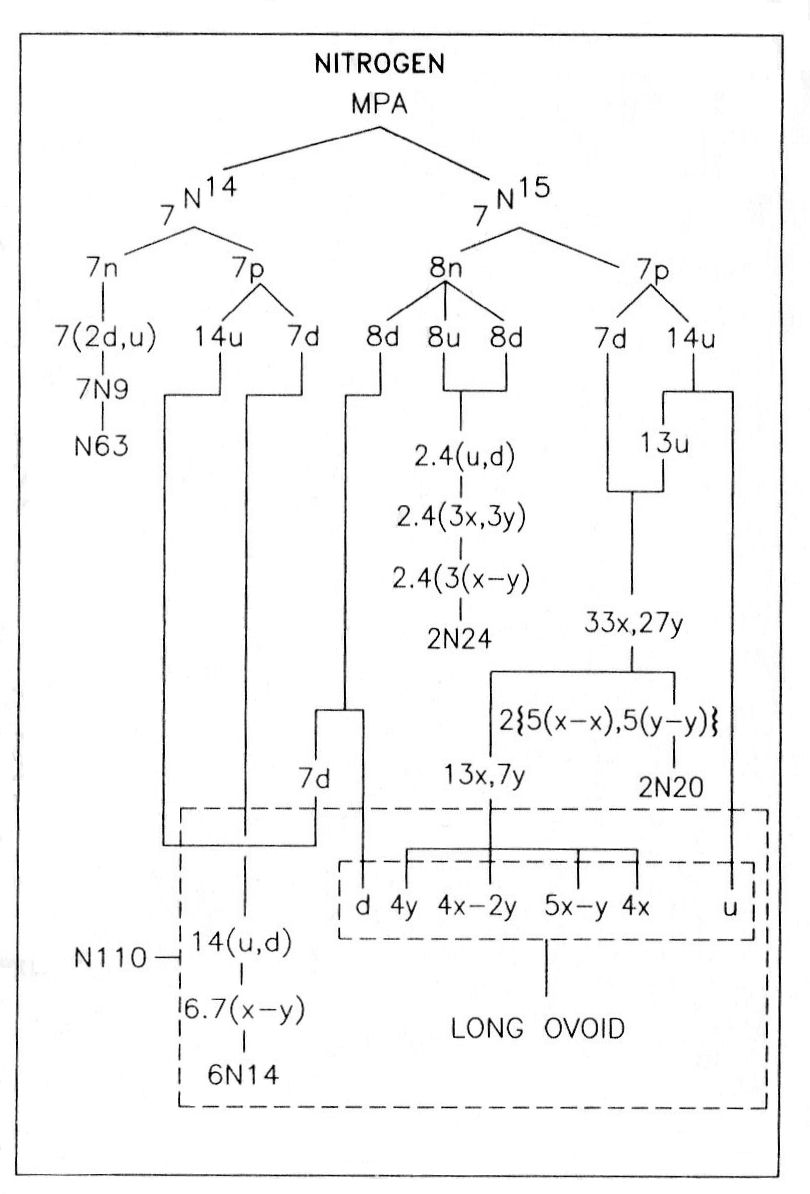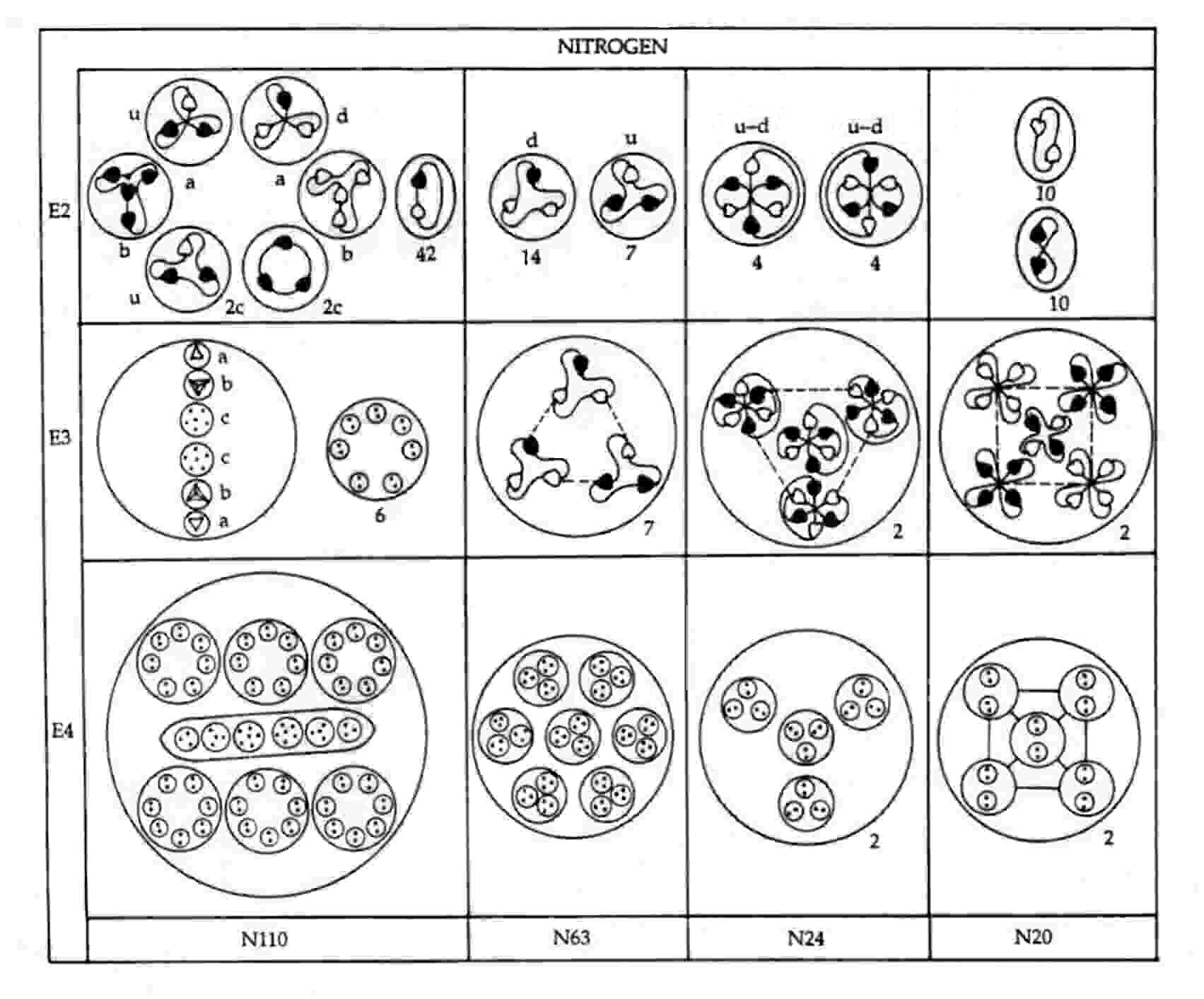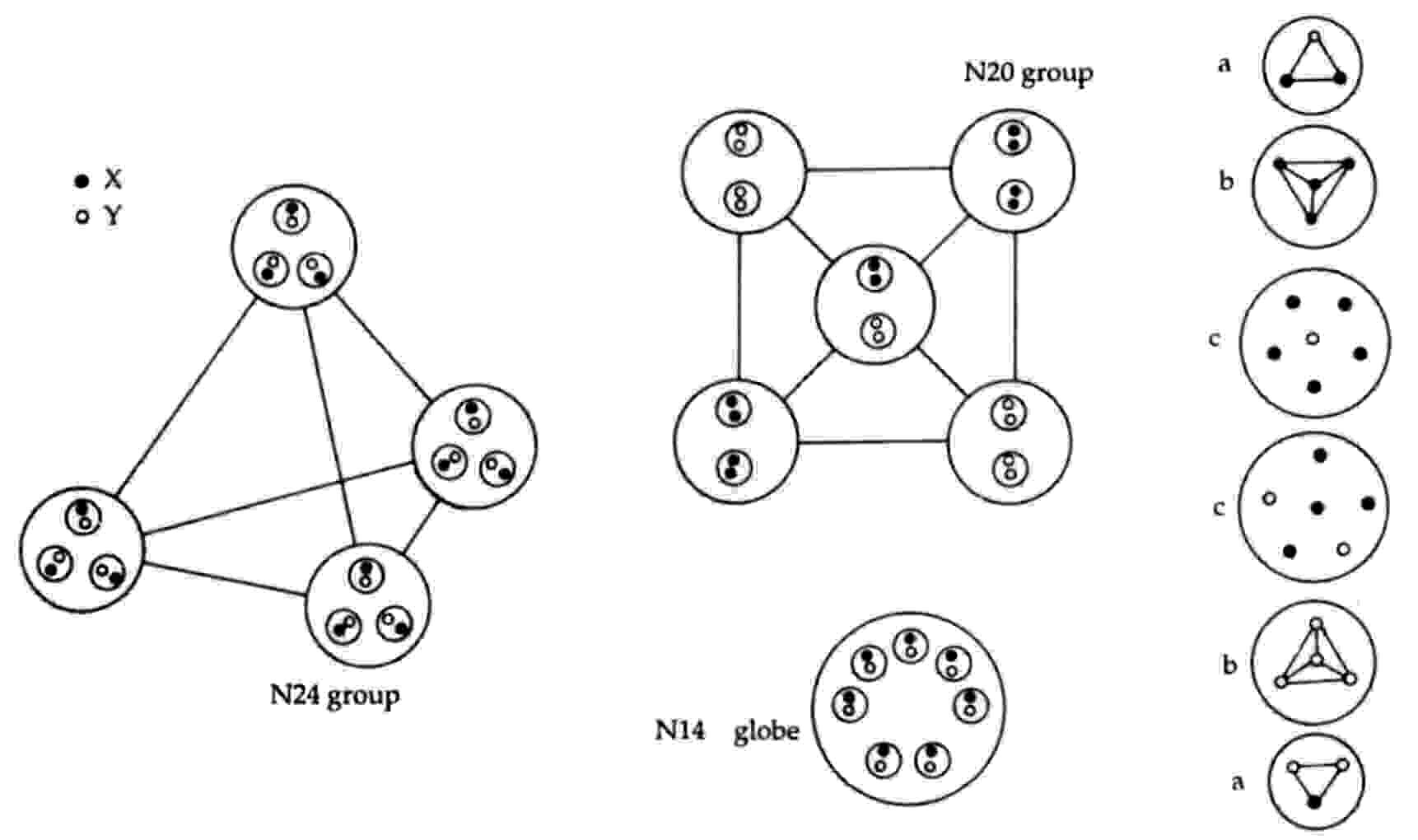The Nitrogen MPAThe sphere-shaped MPA of nitrogen contains six bodies made up of 261 UPAs. A balloon-shaped object (N110) floats in the middle of the sphere, This body is found in the MPAs of many other elements, so that it must be very stable, unable to add or lose particles in a way analogous to how bound states of nucleons forming closed shells determine especially stable nuclides. The N110 contains six smaller spheres arranged in two horizontal rows of three spheres, each one enclosing seven duads of UPAs (N14). It also contains a long ovoid enclosing two triplets, two quartets and two sextets (N6) of UPAs. Below the N110 group is a cluster of seven spheres (N63), each containing three hydrogen triplets (N9). Around the N110 are two "negative" spheres (N20). An N20 contains five spheres, each of which encloses two spheres containing single N2 duads. There are also two "positive" spheres (N24), each enclosing four globes. A globe contains three spheres, each enclosing an N2 duad.

Nitrogen MPA = N110 + N63 + 2N24 + 2N20.

Nitrogen was one of the first elements in the atmosphere to be examined by Besant & Leadbeater in 1895. If its MPA were formed from two N14 nuclei, which contain 252 subquarks, it would contain nine fewer UPAs than what Besant & Leadbeater determined. Nitrogen is one of three elements whose MPAs have UPA populations consistent with their formation from two stable nuclei of the element that differ in mass number by one unit, i.e., a neutron made up of nine subquarks. The relative abundance of the N15 isotope is only 0.365%, compared with 99.635% for N14, making the formation of the nitrogen MPA from the latter far more likely, statistically speaking. However, the description of MPAs by Besant & Leadbeater was not likely based solely on a single investigative session. Indeed, this seems highly implausible, given the complexity of many MPAs. Nor may they have looked at only one MPA of a given element during one session. They may have needed to examine several such MPAs in succession in order to complete their investigation, for their observation of the many types of particles in an MPA would have disturbed it as a whole, necessitating fresh samples. The detailed picture of an MPA is therefore really a pastiche that was probably built up from the use of several samples, not all of which may have formed from the same pair of nuclides. This consideration makes it more plausible that a nucleus of a relatively rare isotope like N15 may have occasionally participated in the formation of the MPA. If the compounded description of the MPA of nitrogen included a contribution exclusively due to the relatively rare N15 nuclide, this would account precisely for its UPA population.

Although entertaining this possibility may sound like special pleading, it is not without further justification. Firstly, an observational error of nine too many UPAs is difficult to explain in terms of smaller errors made during the examination of the various groups making up the MPA. It is highly unlikely that either the N20, N24 or N63 group was wrongly observed because this would destroy the agreement between observation and theory found for many other MPAs containing these groups. The similarity of the seven N14 spheres in the N110 also makes it improbable that they had nine too many UPAs; this being an odd number, at least one sphere would, implausibly, have had to contain a single UPA instead of a duad of UPAs. It would be plausible if the long ovoid in the N110 had been undercounted through UPAs or groups of UPAs being missed. However, it is difficult to see how it could have been overcounted by as many as nine UPAs. Secondly, the assumption that the observed MPA was partly based upon a sample involving nuclei of N14 and N15 accounts for every reported detail, as will be shown next. We do not know how many times Besant & Leadbeater looked for nitrogen, as they built up a picture of its MPA. Given that several samples were involved, it is no longer so improbable that the rarer N15 nuclide entered the formation of a sample nitrogen MPA on one occasion but that the difference it created was not noticed in other samples.The nitrogen MPA as described was formed from an N14 nucleus and an N15 nucleus. Seven neutrons from the former make up the N63 group, an N9 group being a neutron made up of two d quarks and one u quark. This is confirmed by the diagram showing the partlcles released from the MPA. It shows that each N9 in the N63 breaks up at stage E2 into two (−) triplets (d quarks) and one (+) triplet (u quark). The subquarks in eight u quarks and eight d quarks from the eight neutrons in the N15 nucleus regroup to form two clusters of four bound states of the three X-Y bound states:

2N24 = 8u + 8d = 2(4u +4d) = 2[4(2X-Y) + 4(X-2Y)] → 2[4×4(X-Y)].

Mutual Coulombic repulsion between their similar electric charges of +1/3 causes these bound states to have a tetrahedral arrangement, as is confirmed by the observation (referring to each N24 group): "On the E3 level, each assumes a tetrahedral form, with six Anu at each point."1 The disintegration diagram shows that the group of six UPAs rearranges itself into an Ad6 group when the N24 group is broken up. This is possible because the six UPAs are the subquarks making up an u and a d quark and because, according to analyses of many MPAs, an Ad6 group is, usually, an u-d diquark.Subquark composition of particles in the nitrogen MPA.

An N20 group consists of a square pyramidal array of five X-X bound states and five Y-Y bound states. That two types of bound states are present is confirmed by the disintegration diagram, which shows the two N20 groups breaking up at stage E2 into ten (+) duads (X-X) and ten (−) duads (Y-Y).

The N110 group comprises the following particles:

N110 = 6×7(X-Y) + u + 4X-2Y + 5X-Y + 4Y + d.

The first particle is the six N14 globes containing groups of seven duads (X-Y) that surround the long ovoid in the centre of the MPA. The disintegration diagram confirms this identification because it indicates that the N110 releases forty-two (0) duads (X-Y) at stage E2. The other particles make up the long ovoid. The two triplets (a) are a u quark and a d quark, the two quartets (b) are a bound state of four X subquarks and a bound state of four Y subquarks, and the two sextets (c) are a bound state of four X subquarks and two Y subquarks and a bound state of five X subquarks and one Y subquark. The disintegration diagram confirms that the two triplets (a) are a (+) triplet (u quark) and a (−) triplet (d quark). It also indicates that one of the two sextets (c) break up at the E2 stage into two (+) triplets (2c), i.e., two u quarks, which is consistent with its containing four X subquarks and two Y subquarks:

4X-2Y → 2u (= 2X-Y).

The other sextet c breaks up at the E2 stage into a u quark (2X-Y) and a bound state of three X subquarks bound together in a circular string configuration:

5X-Y → 2X-Y + X-X-X

(the two triplets into which it splits (labelled "2c" in the disintegration diagram) do not have the same subquark composition. This prediction cannot be tested because these triplets were not further broken up.

In conclusion, every detail of the nitrogen MPA has been accounted for on the basis that its description was compounded from observations of several sample MPAs, at least one of which happened to be formed from an N14 nucleus and an N15 nucleus instead of two N14 nuclei.

References

1. Occult Chemistry (3rd ed.), p. 173 .

Home